Function Repository Resource:

# HessianPlane

Get the Hessian normal form of a plane passing through three given points in space

Contributed by: Ed Pegg Jr
 ResourceFunction["HessianPlane"][pts] returns the Hessian plane for pts.

## Details

Let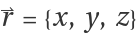and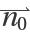be the normal vector {a,b,c}, such that a2+b2+c2=1. The Hessian normal form of a plane is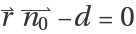, with d the distance from the origin.
The Hessian plane equation a x+b y+c z-d=0 can also be written as {a,b,c,d}.{x,y,z,-1}=0.

## Examples

### Basic Examples (3)

Find the Hessian plane through three points:

 In:=Out=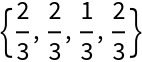The first three components form a unit vector:

 In:=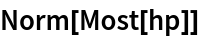Out=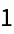Show the plane, points and normal vector:

 In:=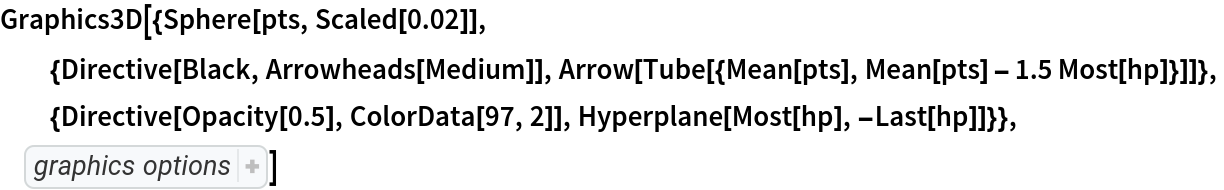Out=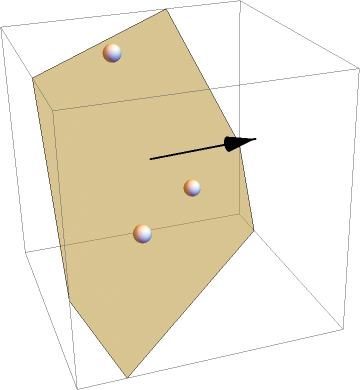### Possible Issues (2)

Timing can be faster than RegionDistance in some cases (though usually it is not):

 In:=In:=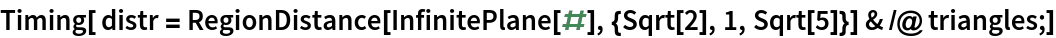Out=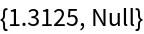In:=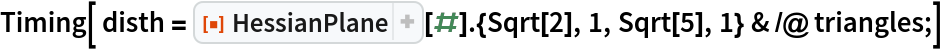Out=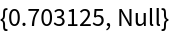In:=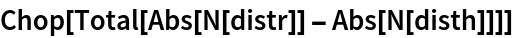Out=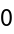HessianPlane fails if the given points do not determine a plane:

 In:=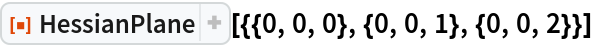Out=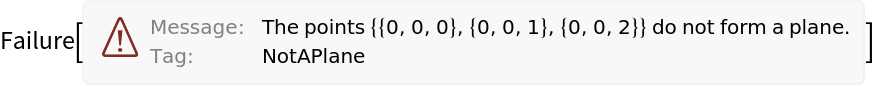### Neat Examples (4)

Points of a random tetrahedron centered at the origin:

 In:=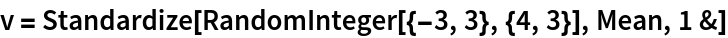Out=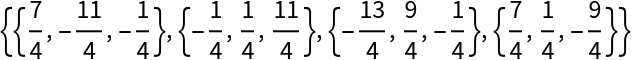Face planes of the tetrahedron:

 In:=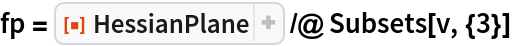Out=Incenters of each face, using the resource function Incircle3D:

 In:=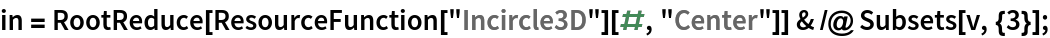Perpendiculars to the incenters do not not intersect, therefore this tetrahedron does not have a midsphere tangent to all edges:

 In:=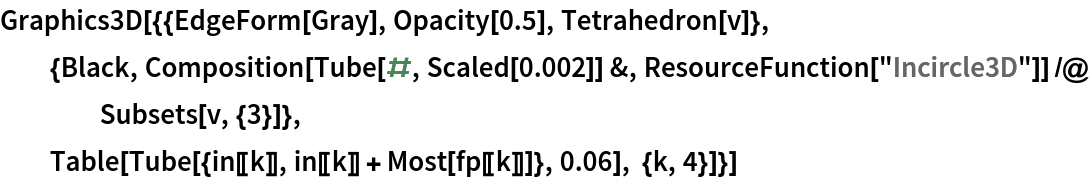Out=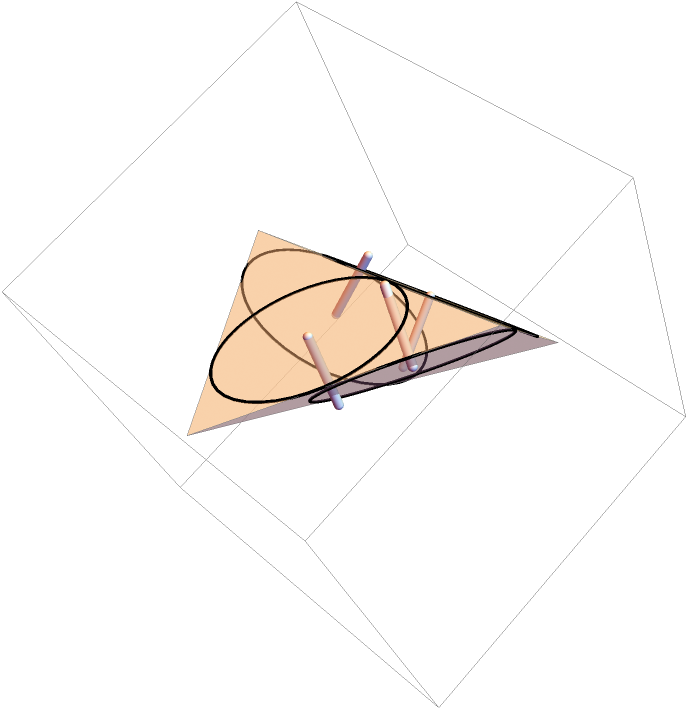## Version History

• 1.0.0 – 31 May 2022

## Author Notes

Not sure if this already exists, but I recreate something like this constantly. If it does exist, then this might be a good function just for pointing at other functions.